Nivethitha

updated on 23-03-2023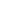# SGPA to Percentage## Convert SGPA to percentage

Percentage

If you are looking for an SGPA to percentage calculator, you can make use of the given SGPA to percentage converter. By entering the SGPA in the given space, you will be receiving the corresponding percentage.

With the advancement in the education system, the universities across the globe are adapting to the grading system of scoring. The calculation methods may differ based on the universities but the SGPA, CGPA, GP, and percentage calculations are mostly the same. You must understand the SGPA to percentage calculation to know how your academic performance is evaluated.

Educational institutions have started using their own SGPA to percentage calculators for their student's convenience. In this article, we will be discussing the SGPA to percentage conversion process, the formula used, the terms involved, CGPA calculation, SGPA calculation for different universities, etc.

Synopsis

## What is SGPA?

The Semester Grade Point Average (SGPA) is used to indicate a student's performance in a year or a semester. SGPA is defined as the weighted average of the grade points secured by the student in each subject registered during the particular semester. The SGPA range differs between 0.00 and 10.00 based on student performance and an SGPA calculator can be used to calculate the value.

SGPA is accepted and used by most educational institutions in India and worldwide. These institutions also use an SGPA to percentage calculator or converter to indicate the student's skill level in percentage. You must perform well in the exams to secure a good SGPA which in turn will lead to a good CGPA.

## How to calculate SGPA?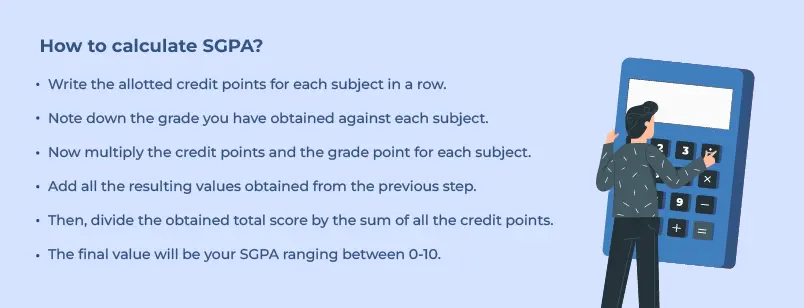To calculate SGPA you must know your grade points obtained per subject, the individual subjects' credit points, and the total number of subjects you have appeared for. The SGPA calculator can be used to easily find the SGPA value. Learning how to calculate SGPA is essential as it reflects on your performance in a particular semester or a year. There are several ways to calculate your SGPA, but let's discuss the simplest way to calculate SGPA.

• Write the allotted credit points for each subject in a row.
• Note down the grade you have obtained against each subject.
• Now multiply the credit points and the grade point for each subject.
• Add all the resulting values obtained from the previous step.
• Then, divide the obtained total score by the sum of all the credit points.
• The final value will be your SGPA ranging between 0-10.

For a better explanation of SGPA calculation, you can follow the given sample of SGPA calculation for a 4 subject semester.

The credit points for the 4 subjects will be as:

• Subject 1 - 3
• Subject 2 - 4
• Subject 3 - 3
• Subject 4 - 5

The grades obtained on these subjects are:

• Subject 1- A
• Subject 2 - O
• Subject 3 - B
• Subject 4 - A+

The following table will show the grade points for the respective grades.

 Marks Range(Out of 100) Description Grade Grade Points 90-100 Outstanding O 10 80-89 Excellent A+ 9 70-79 Very Good A 8 60-69 Good B+ 7 50-59 Above average B 6 40-49 average C+ 5 35-39 Pass P 4 0-34 Fail F 0 Otherwise Absent - -

Now multiple the obtained grades with the credit scores.

• Subject 1 - 8*3 = 24
• Subject 2 - 10*4 = 40
• Subject 3 - 3*6 = 18
• Subject 4 - 5*9 = 45

Next find the summations of the credit scores and the multiplicated values

Credit score sum- 3+4+3+5 = 15
Total score - 24+40+18+45= 127

SGPA = 127/15 = 8.44

You can use the below-given table to understand the calculation better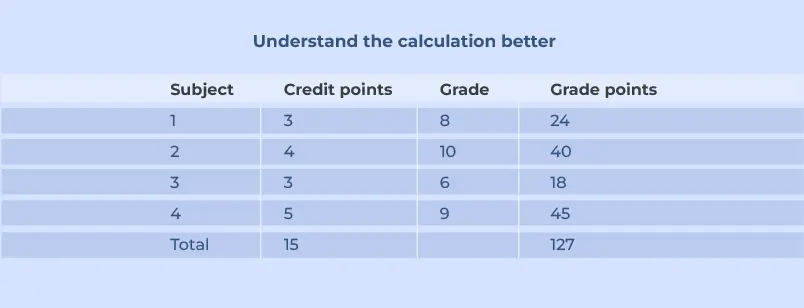SGPA = Sum of grade points / Sum of credit points
= 127/15 = 8.44

## How to convert SGPA into percentage?

The steps followed in converting SGPA to percentage are as follows,

• Calculate the SGPA using the manual calculator or the SGPA calculator.
• Next subtract the SGPA by 0.75
• Multiply the answer by 10.
• If you are calculating your percentage for your complete course duration, you need to add the SGPA for all the semesters and divide the sum by the total number of semesters. The result will be your overall SGPA.

## Formula to convert SGPA to Percentage

To convert SGPA into percentage, you can use the simple formula,

 Percentage = (SGPA - 0.75)*10                      or                       (SGPA *10)-7.5

For instance, if your SGPA is 8.5 then your percentage can be calculated as follows. Subtract 0.75 from 8.5. This would be 7.75. Now multiply 7.75 with 10. Thus your percentage would be 77.5.

Percentage = (SGPA-0.75)*10
= (8.5-0.75)*10 = 77.5%

## What is CGPA?

Cumulative Grade Point Average (CGPA) is the grading system accepted by educational institutions across the globe to offer admission. The CGPA is the educational grading system used to measure a student's performance over an academic tenure. Different countries have their own grading system which can be an alphabet, percentage, number, or range that will indicate the student's educational performance.

The CGPA is obtained by dividing the mean grade points by the number of semesters. The CGPA can be calculated for a particular period of time or an academic year like 1st year or 2nd year.

The table below may give an insight into the basic CGPA calculation based on percentage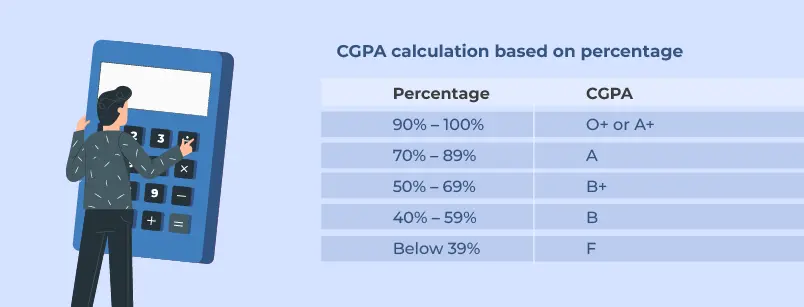## How to convert SGPA to CGPA?

The SGPA being the cumulative grade point of a particular semester, the CGPA can be easily calculated from the SGPA. The mean value of the SGPA over a period of education gives you the CGPA.

For instance, if the SGPA for 3 semesters is 7, 8, and 5, then the CGPA will be calculated as follows.

CGPA = (Total sum of SGPA) / total number of semesters
= (7+8+5)/(3)
= 6.66

## Difference between SGPA and CGPA

The main difference between the SGPA and CGPA is that SGPA is the mean value of the grade points obtained per semester, whereas CGPA is the cumulative grade points obtained in an academic year. The sum of SGPA will give the CGPA. Thus for a good CGPA, you must have a perfect SGPA each semester. Both terms are used to reveal the student's academic performance.

### Common terms and meanings used in SGPA to percentage calculation

To understand how to calculate percentages from SGPA, you must be aware of the terms involved in the SGPA calculation. Knowing these terms will help you in cross-checking your values, even after using the SGPA to percentage calculator. The terms are as follows,

#### Semester

The course tenure of your education is divided into small segments known as semesters. In one academic year, there will be two semesters. For instance, in engineering courses, there are a total of 8 semesters in 4 years.

#### Credit points

The study load of a subject is measured as credit points. The credit points will be assigned by the universities based on the hours spent on the particular course during the semester. The educational system assesses your progress and completion of the course based on the credit points received.

You will be awarded a grade based on the marks obtained in that particular subject. Each grade will be having its corresponding grade points with which the overall performance of the student in a particular subject will be determined.

## How to calculate SGPA from marks?

• If marks are given, write the marks and note down the corresponding grades.
• Now multiply the grade points by the corresponding subjects’ credit points.
• To calculate the SGPA, divide the total grade points by the sum of all the credit points.
• To obtain the percentage from SGPA, you can use the formula (SGPA-0.75)*10.

## How to calculate SGPA in engineering?

The SGPA for an engineering course is calculated using credit points and grade points. Usually, the engineering results are represented in SGPA, CGPA, and percentage. The SGPA will be calculated per semester and the CGPA will be calculated for the entire academic tenure. The SGPA to percentage converter can be used to calculate the student's performance in percentage.

## SGPA calculator for different Indian universities

The performance measurement unit adopted by Indian universities to elevate the students’ academic achievement is SGPA. There are a number of ways to calculate the SGPA and convert SGPA to percentage. Thus we have listed a few of the top universities' SGPA for percentage calculation for your reference.

## SGPA to percentage SPPU conversion

Savitribai Phule Pune University follows a 10-point grade system. The SPPU results will be represented using the SGPA and CGPA. The SGPA to percentage SPPU calculation is as follows,

SGPA = (Sum of (grade point * credit points))/ (sum of the credit points)

CGPA = (Sum of the SGPA )/ (Total number of semesters)

SGPA to percentage = (SGPA*10)- 7.5

CGPA to percentage = (CGPA*8.8)

## SGPA to percentage calculator in AKTU

Abdul Kalam Technical University, uses SGPA, YGPA, and CGPA. The individual scores will be calculated as SGPA and the Yearly Grade Points Average will be the grade points obtained per year. The CGPA will be calculated at the end of the last semester when the overall SGPA and YGPA will be known.

 SGPA (Si) = Σ (Ci x Gi) / ΣCi

Where
Ci - will be the credit points
Gi - will be the grade points

## SGPA calculation in VTU

Visvesvaraya Technological University, Belagavi follows the choice-based credit system to assess the student’s performance. The transcripts or the grade card provided by the VTU will contain the terms SGPA, GPA, and CGPA. The SGPA to percentage VTU calculation will provide you with the percentage of marks obtained by you.

Here the SGPA can be calculated as,

SGPA - (Ci1*Gi1+ Ci2*Gi2+ Ci3*Gi3)/ (Ci1+Ci2+Ci3)
Where,
Ci1, Ci2, and Ci3 are the credit points of subject 1, subject 2, and subject 3.
Gi1, Gi2, and Gi3 are the grade points for subjects 1, subject 2, and subject 3.

## How to calculate SGPA in JNTUH

Jawaharlal Nehru Technological University, Hyderabad, used an absolute 10-point grade system to indicate the student's performance in their semester, project work, yearly exam, etc. The students have to earn sufficient Grade Points (GP) in order to clear the subject. The table below will showcase the percentage of marks obtained, corresponding grades, and their GP according to the UGC guidelines.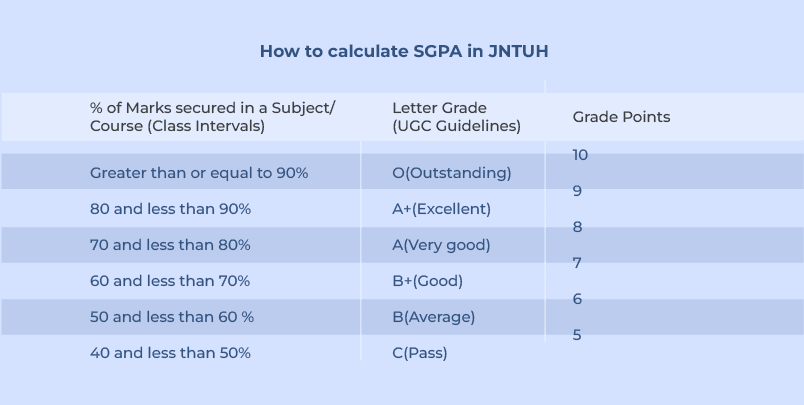## How to calculate SGPA in Mumbai university

The University of Mumbai is using two types of grading system, a 7-point grading system, and 10 point grading system. For the 7-point grading system (CBGS), the percentage will be calculated based on the actual marks obtained by the students in each subject. The percentage of the 10-point grading system will be calculated using the following formula,

 Percentage = 7.1* CGPA +11

Thus the SGPA to percentage Mumbai University conversion process is as follows. First the individual semester SGPA will be calculated, then CGPA will be derived using the SGPA and finally converted into percentages using the formula.

## How to convert SGPA to CGPA to Percentage?

For easy understanding, here you will be seeing how the SGPA to percentage calculation works from an example.

Semester 1:

 Subject Grade Grade points(GP) Credit points(CP) GP*CP 1 A+ 9 3 27 2 B+ 7 3 21 3 O 10 4 40 4 C 5 3 15 Total 13 103

SGPA for semester 1 = (GP*CP)/ (Sum of CP)
= 103/13 = 7.92

SGPA to percentage for Semester 1= (SGPA *10)- 7.5
= (7.92*10)-7.5 = 71.7%

Semester 2:

 Subject Grade Grade Points(GP) Credit Points GP*CP 1 A 8 4 32 2 A+ 9 4 36 3 B 6 2 12 4 B+ 7 2 14 Total 12 94

SGPA for semester 2 = (GP*CP)/ (Sum of CP)
= 94/12 = 7.83

SGPA to percentage for Semester 1= (SGPA *10)- 7.5
= (7.83*10)-7.5 = 70.83 %

CGPA = (SGPA for semester 1 + SGPA for Semester 2) / 2
= (7.92+7.83) / 2
= 7.875

## SGPA to percentage conversion chart

 SGPA PERCENTAGE 10 92.5% 9.9 91.5% 9.8 90.5% 9.7 89.5% 9.6 88.5% 9.5 87.5% 9.4 86.5% 9.3 85.5% 9.2 84.5% 9.1 83.5%

 SGPA PERCENTAGE 9.0 82.5% 8.9 81.5% 8.8 80.5% 8.7 79.5% 8.6 78.5% 8.5 77.5% 8.4 76.5% 8.3 75.5% 8.2 74.5% 8.1 73.5%

 SGPA PERCENTAGE 8.0 72.5% 7.9 71.5% 7.8 70.5% 7.7 69.5% 7.6 68.5% 7.5 67.5% 7.4 66.5% 7.3 65.5% 7.2 64.5% 7.1 63.5%

 SGPA PERCENTAGE 7.0 62.5% 6.9 61.5% 6.8 60.5% 6.7 59.5% 6.6 58.5% 6.5 57.5% 6.4 56.5% 6.3 55.5% 6.2 54.5% 6.1 53.5%
 SGPA PERCENTAGE 6.0 52.5% 5.9 51.5% 5.8 50.5% 5.7 49.5% 5.6 48.5% 5.5 47.5% 5.4 46.5% 5.3 45.5% 5.2 44.5% 5.1 43.5%

 SGPA PERCENTAGE 5.0 42.5% 4.9 41.5% 4.8 40.5% 4.7 39.5% 4.6 38.5% 4.5 37.5% 4.4 36.5% 4.3 35.5% 4.2 34.5% 4.1 33.5%

## FAQ for SGPA to Percentage conversion

### 1. How much is 7.5 SGPA in Percentage?

The SGPA can be easily converted into a Percentage using the formula,
Percentage - (SGPA-0.75)*10

= (7.5-0.75)*10
= 67.5 %

### 2. How is SGPA of one-semester percentage calculated?

The first step is to calculate the SGPA by multiplying the grade points by the credit points of the individual subjects. Add all the final values and derive the sum of total grade points. The SGPA can be arrived at by dividing the total grade points by the summation of the credit points.
The SGPA to percentage conversion is done using the usual formula of multiplying the SGPA by 10 and then subtracting the result by 7.5.

### 3. What is a good SGPA?

Usually, an SGPA above 8.0 is considered a good score. Having a persistent academic performance and having SGPAs above 8 in all semesters will lead to a good CGPA and in turn a good percentage.

### 4. Are SGPA and CGPA the same?

No, the SGPA and CGPA are not the same. Though they may look identical, the terms differ based on their calculating period. The SGPA is calculated per semester and the CGPA is calculated for the overall education tenure.

### 5. What is the maximum SGPA?

SGPA is the measure of the student's performance in a semester. The SGPA is ranging from 0.00 to 10.00 with 10.00 being the maximum SGPA value. The value will be represented with two decimal points.Nivethitha is an experienced IELTS trainer based in Chennai, who has helped over 1000+ students achieve their desired band scores and gain admission to their dream universities. With over 3 years of experience and a strong educational background in English literature, she is an expert in the language and its nuances. She holds both TEFL and Business English communication certifications, further enhancing her skills and knowledge in the field. Nivethitha believes in the importance of practice tests and incorporates them heavily into her training to help boost her students' confidence and skillset. She aims to provide practical tips and actionable advice to make the aspirants achieve their academic and career goals through her trusted authority in the field of IELTS training.

More Articles Post by Nivethitha

Kanan International is an IRCC-certified global education partner that offers reliable services, products, and solutions for students and education institutions. We support students throughout their study abroad journey, ensuring a hassle-free experience. Our expertise and commitment to excellence make us the go-to partner for expanding global horizons. The company provides coaching for IELTS, GRE, TOEFL, and SAT exams and services like visa consultation, accommodation, scholarship assistance, and education loans.

#### Recent posts from NivethithaNivethitha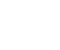Nivethitha

SGPA to PercentageNivethitha

CGPA to Percentage Conversion | CBSE Formula & CalculatorNivethitha

Education loan in USA for Indian studentsNivethitha

Best place to study in CanadaNivethitha

IELTS Band ScoreA truly global higher education partner to learners and education institutions Support learners at every point in their global education journey with reliable services, products and solutions.

Kanan Int EdTech Inc

229, Yonge Street Suite 406 Toronto Ontario, Canada M5B 1N9

Kanan International Pvt. Ltd.+91 63570 12000admissions@kanan.co